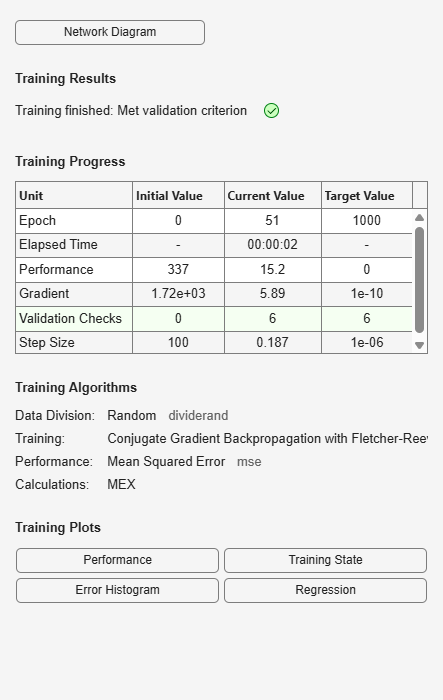# traincgf

## Syntax

```net.trainFcn = 'traincgf' [net,tr] = train(net,...) ```

## Description

`traincgf` is a network training function that updates weight and bias values according to conjugate gradient backpropagation with Fletcher-Reeves updates.

`net.trainFcn = 'traincgf'` sets the network `trainFcn` property.

`[net,tr] = train(net,...)` trains the network with `traincgf`.

Training occurs according to `traincgf` training parameters, shown here with their default values:

 `net.trainParam.epochs` `1000` Maximum number of epochs to train `net.trainParam.show` `25` Epochs between displays (`NaN` for no displays) `net.trainParam.showCommandLine` `false` Generate command-line output `net.trainParam.showWindow` `true` Show training GUI `net.trainParam.goal` `0` Performance goal `net.trainParam.time` `inf` Maximum time to train in seconds `net.trainParam.min_grad` `1e-10` Minimum performance gradient `net.trainParam.max_fail` `6` Maximum validation failures `net.trainParam.searchFcn` `'srchcha'` Name of line search routine to use

Parameters related to line search methods (not all used for all methods):

 `net.trainParam.scal_tol` `20` Divide into `delta` to determine tolerance for linear search. `net.trainParam.alpha` `0.001` Scale factor that determines sufficient reduction in `perf` `net.trainParam.beta` `0.1` Scale factor that determines sufficiently large step size `net.trainParam.delta` `0.01` Initial step size in interval location step `net.trainParam.gama` `0.1` Parameter to avoid small reductions in performance, usually set to `0.1` (see `srch_cha`) `net.trainParam.low_lim` `0.1` Lower limit on change in step size `net.trainParam.up_lim` ` 0.5` Upper limit on change in step size `net.trainParam.maxstep` `100` Maximum step length `net.trainParam.minstep` `1.0e-6` Minimum step length `net.trainParam.bmax` `26` Maximum step size

## Network Use

You can create a standard network that uses `traincgf` with `feedforwardnet` or `cascadeforwardnet`.

To prepare a custom network to be trained with `traincgf`,

1. Set `net.trainFcn` to `'traincgf'`. This sets `net.trainParam` to `traincgf`’s default parameters.

2. Set `net.trainParam` properties to desired values.

In either case, calling `train` with the resulting network trains the network with `traincgf`.

## Examples

collapse all

This example shows how to train a neural network using the `traincgf` train function.

Here a neural network is trained to predict body fat percentages.

```[x, t] = bodyfat_dataset; net = feedforwardnet(10, 'traincgf'); net = train(net, x, t);````y = net(x);`

collapse all

### Conjugate Gradient Algorithms

All the conjugate gradient algorithms start out by searching in the steepest descent direction (negative of the gradient) on the first iteration.

`${p}_{0}=-{g}_{0}$`

A line search is then performed to determine the optimal distance to move along the current search direction:

`${x}_{k+1}={x}_{k}{\alpha }_{k}{p}_{k}$`

Then the next search direction is determined so that it is conjugate to previous search directions. The general procedure for determining the new search direction is to combine the new steepest descent direction with the previous search direction:

`${p}_{k}=-{g}_{k}+{\beta }_{k}{p}_{k-1}$`

The various versions of the conjugate gradient algorithm are distinguished by the manner in which the constant βk is computed. For the Fletcher-Reeves update the procedure is

`${\beta }_{k}=\frac{{g}_{k}^{T}{g}_{k}}{{g}_{k-1}^{T}{g}_{k-1}}$`

This is the ratio of the norm squared of the current gradient to the norm squared of the previous gradient.

See [FlRe64] or [HDB96] for a discussion of the Fletcher-Reeves conjugate gradient algorithm.

The conjugate gradient algorithms are usually much faster than variable learning rate backpropagation, and are sometimes faster than `trainrp`, although the results vary from one problem to another. The conjugate gradient algorithms require only a little more storage than the simpler algorithms. Therefore, these algorithms are good for networks with a large number of weights.

Try the Neural Network Design demonstration `nnd12cg` [HDB96] for an illustration of the performance of a conjugate gradient algorithm.

## Algorithms

`traincgf` can train any network as long as its weight, net input, and transfer functions have derivative functions.

Backpropagation is used to calculate derivatives of performance `perf` with respect to the weight and bias variables `X`. Each variable is adjusted according to the following:

```X = X + a*dX; ```

where `dX` is the search direction. The parameter `a` is selected to minimize the performance along the search direction. The line search function `searchFcn` is used to locate the minimum point. The first search direction is the negative of the gradient of performance. In succeeding iterations the search direction is computed from the new gradient and the previous search direction, according to the formula

```dX = -gX + dX_old*Z; ```

where `gX` is the gradient. The parameter `Z` can be computed in several different ways. For the Fletcher-Reeves variation of conjugate gradient it is computed according to

```Z = normnew_sqr/norm_sqr; ```

where `norm_sqr` is the norm square of the previous gradient and `normnew_sqr` is the norm square of the current gradient. See page 78 of Scales (Introduction to Non-Linear Optimization) for a more detailed discussion of the algorithm.

Training stops when any of these conditions occurs:

• The maximum number of `epochs` (repetitions) is reached.

• The maximum amount of `time` is exceeded.

• Performance is minimized to the `goal`.

• The performance gradient falls below `min_grad`.

• Validation performance (validation error) has increased more than `max_fail` times since the last time it decreased (when using validation).

## References

Scales, L.E., Introduction to Non-Linear Optimization, New York, Springer-Verlag, 1985

## Version History

Introduced before R2006a